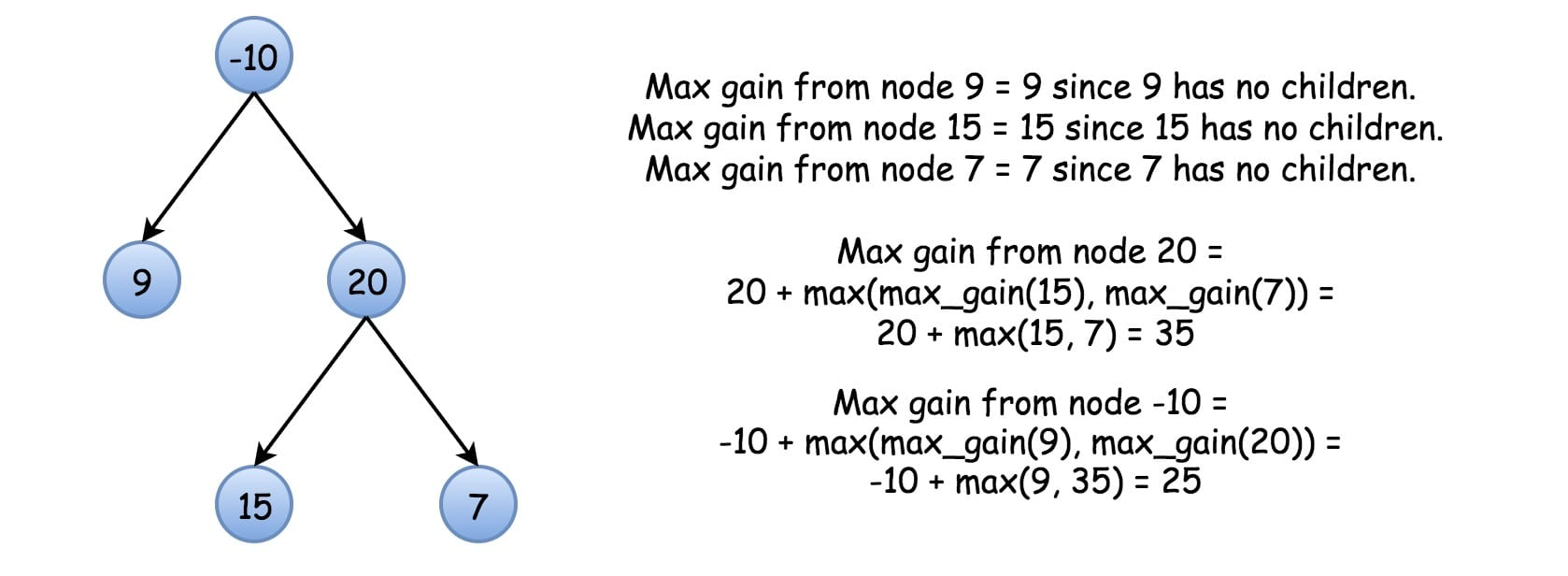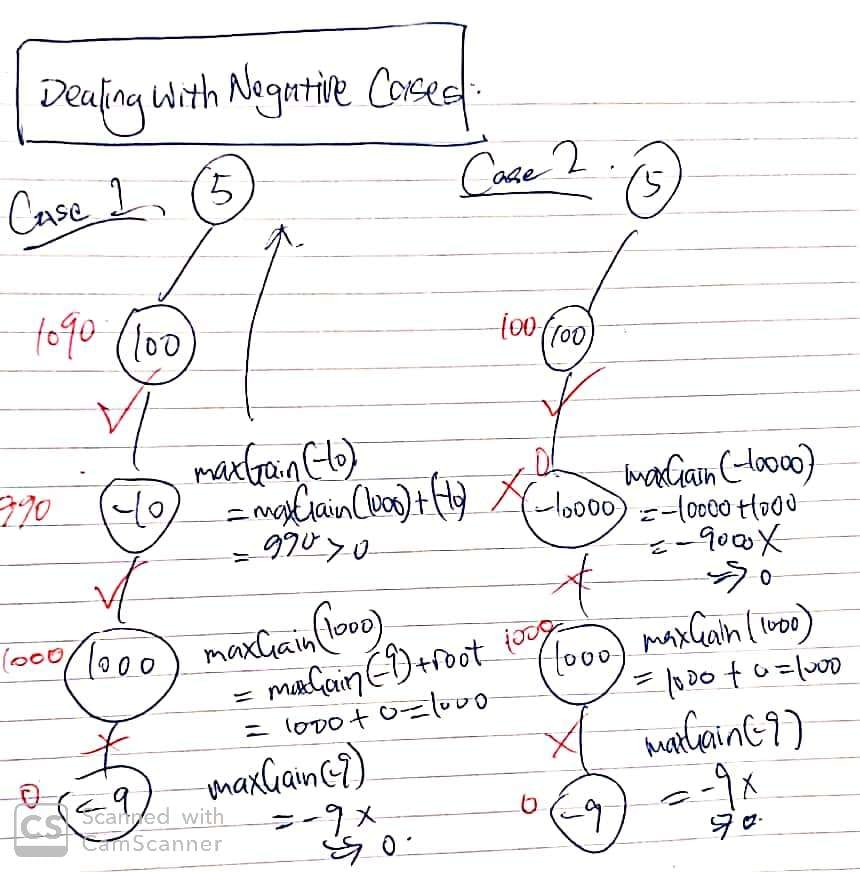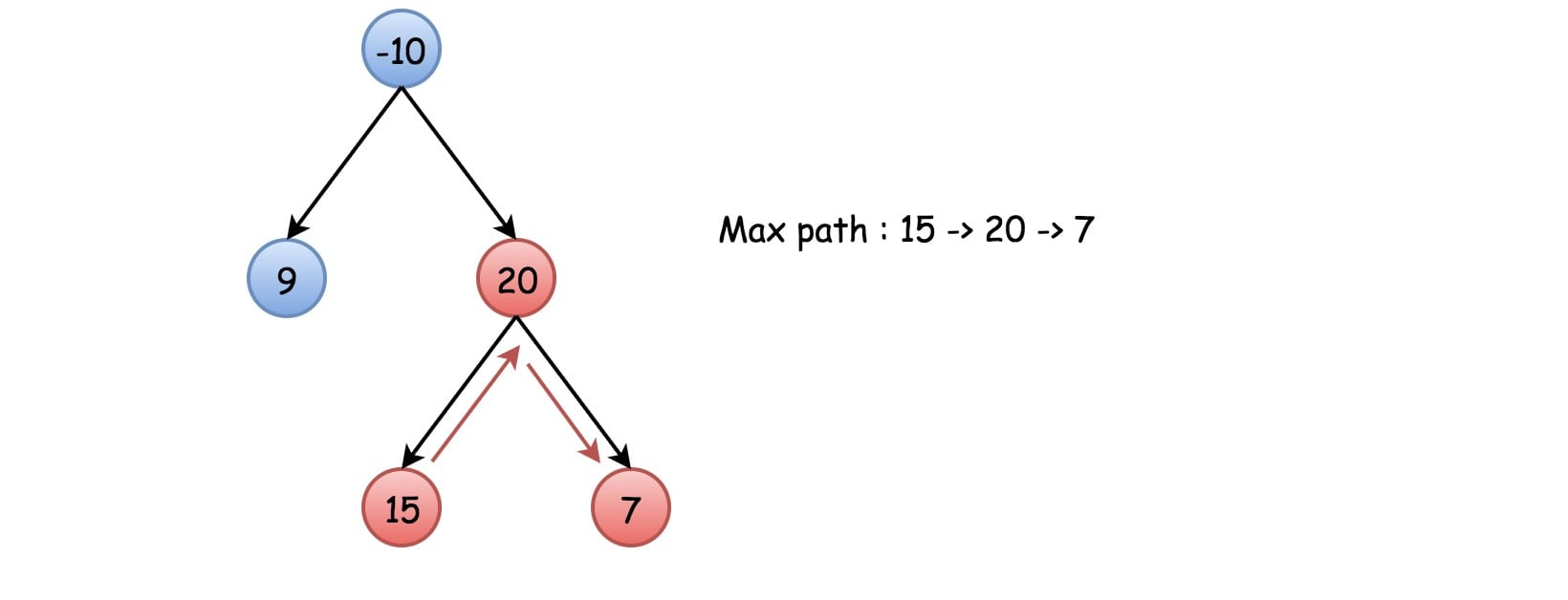# 124. Binary Tree Maximum Path Sum

Reference: LeetCode
Difficulty: Hard

## Problem

Given a non-empty binary tree, find the maximum path sum.

For this problem, a path is defined as any sequence of nodes from some starting node to any node in the tree along the parent-child connections. The path must contain at least one node and does not need to go through the root.

Example:

Also, notice the following cases:

## Analysis

Methods:

First, let’s understand the idea of maxGain(node).Most importantly, we need to design a maxGain(node) that can handle negative numbers. The idea is as follows:However, just using maxGain(node) could not give us the correct result, because the max sum path does not necessarily go through node. So we can use a field to store this value.1. Recursion
• This problem could be simplified by implementing a function maxGain(node) which computes the maximum path sum from this node to one node below (it could be a non-leaf node). Here are the rules inside maxGain(node)
• Root must be used.
• At most one child can be used, or not used when both have negative gains.
• If one would know that the max sum path contains node, the problem would be solved as maxGain(node). However, this path does not necessarily go through the node. A max sum path could be a path at the bottom not crossing the node.
• Thus, we need to modify the function and to check which path is better and update the maximum value if necessary (a field variable). As for maxGain(node), it still returns the maximum gain of the path that crosses the node.
• Note: The idea is not easy, but not hard to understand. However, how to manage the design of recursive functions and handle corner cases is super tricky and complicated if not using the trick (int leftGain = Math.max(maxGain(node.left), 0)).
• Time: $O(N)$ since we visit each node for no more than $2$ times.
• Space: $O(h)$

## Code

### Original Version

Note: 140 ms

• The time complexity is $O(N^2)$ in the worst case, since it does a lot of repeated calculations.
• It just separate the checking idea from the maxGain(node) and make it into maxPathSum(node). Actually, it turns out that we could do it in one function.

### Initial Improvement

Note: 2 ms

• The reason why this code is too long and complicated is because I check a lot of corner cases for negative numbers. For example:
• [-3] should return -3 rather than 0. root == null will lead to return 0, but it should not be treated as a valid return value because it is not a node at all. In other words, 0 should not be compared with -3.
• [2, -1] should return 2 rather than 2 + (-1) = 1.
• Therefore, when calculating the max sum, I have to do many combinations of the results of root.val, root.left, root.right.

### Clean Solution

Note: 1 ms

• Learn how to calculate the maximum values among several values. It turns out that if maxGain(node) returns a negative number, we would definitely not to choose it as our part of values. With this simple code, it handles all the cases as you can imagine.
• If the maxGain(node) of a node’s left or right child is negative, we will immediately drop that value and start over from node.

CommentJunhao Wang
a software engineering cat